# Fundamental Frequency And Harmonics: What Are They?

Contents

## What Are Harmonics?

Harmonics are defined as an unwanted higher frequency component that is an integer multiple of the fundamental frequency. Harmonics create a distortion in the fundamental waveform.

Harmonics usually have a lower amplitude (volume) than the fundamental frequency.

### What is Amplitude?

The maximum value (positive or negative) of an alternating quantity is known as its amplitude.

### Sources of Harmonics

Harmonics are produced due to the non-linear loads such as an iron-cored inductor, rectifiers, electronic ballasts in fluorescent lights, switching transformers, discharge lighting, saturated magnetic devices and other such loads that are highly inductive in nature.

Harmonics are also due to the most powerful electronic switching circuits such as a silicon controlled rectifier (SCR), the power transistors, the power converters, and an electronics drive such as the variable frequency drive (VFD) or variable voltage variable frequency drive (VFD). These switching circuits draw a current only at the peak values of the AC supply and since the switching current is non-linear in behavior the load current is non-sinusoidal in nature and it contains the harmonics.

### What Are the Effects of Harmonics?

Harmonic frequencies in the power grid cause power quality problems.

Harmonics in power systems result in increased heating in the equipment and conductors and create a pulsating torque in the motors.

Harmonics cause increasing operating temperature and the iron losses (Hysteresis and Eddy current losses) in the AC motors and transformers because hysteresis loss is proportional to the frequency and eddy current loss is proportional to the square of the frequency.

Harmonics create misfiring in the variable speed drives since harmonics are higher than fundamental frequencies and at a fundamental frequency the AC machines have a particular speed called a synchronous speed( Ns=120f/P) so at a higher frequency we got a speed difference i.e. slip difference.

In an induction motor crawling phenomenon occurs due to the space harmonics produced by the winding currents.

#### What Are Time Harmonics and Space Harmonics?

The harmonics which are generated by a source that varies non-sinusoidal in time which is always present in the input supply are known as time harmonics.

The harmonics which are a result of the non-sinusoidal distribution of the coils in the machine and slotting, due to that the air-gap mmf and flux are not sinusoidally distributed in space are known as space harmonics.

## What is Fundamental Frequency?

The lowest or base frequency produced by any particular instrument which we hear the sound at is known as the fundamental frequency. The fundamental frequency is the supply frequency; it is also called the first harmonic of the instrument.

The number of cycles completed by an alternating quantity per second is known as a frequency. It is denoted by f and expressed in hertz (Hz) or cycles/second.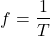## How to Find Fundamental Frequency

In a simple 2-pole generator, one cycle of the alternating current or voltage is generated in one revolution. In the case of 4 poles, two cycles would have been produced in each revolution. So, the frequency of the alternating voltage or current is given by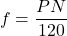Where:

• f = Frequency
• P = No. of poles of the alternator
• N= Speed of the alternator in rpm

The standard frequency of generation adopted in India is 50Hz while in the USA, it is 60Hz.

## How to Calculate Harmonics

Harmonics are the sinusoidal voltage or current that is an integer (whole number) at a multiple of the fundamental frequency at which the supply system is designed to operate.

It can be expressed as a 2f, 3f, 4f, 5f,….……,nf, etc.

So if 50Hz fundamental is the first harmonic then 2nd harmonic would be 100Hz (250), a 3rd harmonic would be 150Hz (350), a 5th harmonic at 250Hz, a 7th at 350Hz and so on.

### Harmonics Calculation Formula

1st Harmonic: It is a fundamental frequency.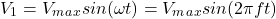2nd Harmonic: It is two times the fundamental frequency.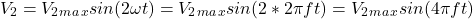3rd Harmonic: It is three times the fundamental frequency.4th Harmonic: It is four times the fundamental frequency.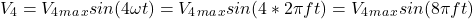And for

nth Harmonic: It is n times the fundamental frequency.So the value of complex waveform (i.e. fundamental plus harmonics) will be: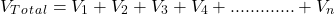## Types of Harmonics

Classifications of harmonics are generally based on their name and frequency. For example, at a fundamental frequency, the 2nd harmonic frequency is 100Hz. Harmonic sequence related to the phasor rotation of the harmonics voltages and currents to the fundamental waveform in a balanced system.

Based on a sequence there are three types of sequence harmonics.

Positive sequence harmonic: A positive sequence harmonic would rotate in the forward (same) direction as that of the fundamental frequency. i.e. 4th, 7th, 10th, 13th, 16th …etc

Negative sequence harmonic: A negative sequence harmonic rotates in the reverse (opposite) direction of the fundamental frequency. i.e. 2nd, 5th, 8th, 11th …etc

Zero sequence harmonics: A zero sequence harmonic does not rotate with the fundamental hence it has zero rotational sequences and they are in phase with each other. It is a set of special harmonics called “triplens” (multiple of three). Triplens are multiples of the third harmonic. i.e. 3rd, 6th, 9th, 12th, 15th …etc

### Effects of Positive, Negative and Zero Sequence Harmonics

Due to a positive sequence harmonic the overheating of conductors, power lines and transformer takes place because of addition to the fundamental as it’s rotating in forward (same) direction as the fundamental frequency.

Negative sequence harmonic circulates negative sequence current between the phases which causes the opposite phasor rotation and due to that the rotating magnetic field may be weakened and thus mechanical torque is reduced especially in the induction motors.

Zero sequence currents circulate between the phase and neutral which add up arithmetically in the common neutral wire and the neutral current becomes three times the phase current causing it to become less efficient and overheat.

### Current Harmonics

In the AC system, the alternating current varies sinusoidally at a particular frequency called the fundamental frequency, usually 50Hz or 60Hz.

Current Harmonics are caused by the non-linear loads such as switching transformers, discharge lighting, saturated magnetic devices, electronic ballasts in fluorescent lights, computer and data-processing loads, laser printers, half-wave rectifiers, SMPS, PLC, refrigerators, IGBTs, MOSFETs, etc.. in which current is not proportional to the applied voltage.

The effect of these loads is the distortion of the fundamental sinusoidal current waveform and the current waveform becomes quite complex.

A Linear electrical load is one in which the current at any time is proportional to the applied voltage. It draws the current at the same frequency as the voltage.

A Non-Linear load is one in which the current is not proportional to the applied voltage. It does not draw the current at the same frequency as the voltage.

#### Effects of Current Harmonics

Harmonic currents increase I2R heat losses in the transformer winding. If harmonic current has supplied to the transformer, the less fundamental current it can provide for powering the loads because harmonic current doesn’t deliver any power so it reduces the number of loads that can be powered.

Due to the additive nature of the 3rd harmonic (triplens) the currents in a phase-to-neutral connected load cause a sharp increase in the zero-sequence current and therefore increases the current in the neutral conductor, being three times the fundamental frequency.

Current harmonics are mostly caused by the non-linear loads but the linear load will also generate current harmonics if the supply voltage waveform is significantly distorted.

The second major effect of the current harmonics on an electrical system is the creation of the voltage harmonics.

### Voltage Harmonics

Voltage harmonics are generated by the current harmonics which distort the voltage waveform. Current distortion interacts with the system impedance creating the voltage distortion.

The non-linear loads generate currents that are rich in harmonic frequency components that generate harmonic voltages.

#### Effects of Voltage Harmonics

The harmonic voltage causes increased eddy current losses in the motors and transformers and it has a significant effect on the operating temperature. Harmonic voltages in a stator induce high-frequency currents in the rotor further increase losses.

The negative sequence 5th harmonic voltage when supplied to an induction motor produces a negative torque which drives the motor in a reverse direction and slows down its rotation. The result is overcurrent in the motor.

Harmonic voltages can produce excessive vibration in both the single and three-phase motors. Vibration leads to higher than normal wear and tear on the bearings.

### Odd and Even Order Harmonics

The harmonics occurring at a frequency 3f, 5f, 7f, 9f…etc are called the odd-order harmonics. The harmonics occurring at a frequency 2f, 4f, 6f, 8f…etc are called the even-order harmonics.

In even order harmonics there is an equal number of positive and negative half-cycles so they cancel out and not significant in power system. While in case of odd harmonics there is a positive half cycle left in each order (e.g. in 3rd order odd harmonics contains two positive cycles and one negative cycle, 5th order odd harmonic contains three positive cycles and two negative cycles) which does not cancel the negative half cycle, therefore it is significant in power system which add up and increase the neutral current and temperature rises.

The odd-order harmonics are mostly produced by the non-linear loads. The even-order harmonics are produced when the uneven current is drawn between the positive and negative half cycle of the fundamental frequency.

The electric arc furnaces, arc welders generate non-integer harmonics. Transformer magnetizing currents contain even order harmonic.

## Difference Between Harmonics and Overtones

The differences between harmonics and overtones have been summarised the table below:

 Harmonics Overtones A harmonic is an integral multiplication of the fundamental frequency. An overtone is defined as any frequency which is greater than the fundamental frequency. Harmonics starts counting from the fundamental frequency. Overtones start counting after the fundamental frequency and starts counting from the harmonics. All harmonics are stationary waves. Overtones may be stationary not stationary waves. Those overtones which match the frequencies of the harmonic acts as a stationary wave. Harmonics are a resonant frequency. Overtones are also a resonant frequency. Example.The first harmonic is a Fundamental frequency (f). Second harmonic is two times the fundamental frequency (2f). Example. Second harmonic (2f) is the first overtone. Third harmonic (3f) is the second overtone.

Want To Learn Faster? 🎓
Get electrical articles delivered to your inbox every week.
No credit card required—it’s 100% free.